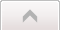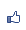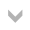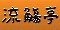流觴亭 市長： 煙 　副市長： 逍遙人 、觴-酒寒 、‧新月‧ 、李小花 加入本城市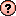｜推薦本城市｜加入我的最愛｜訂閱最新文章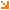本城市首頁討論區精華區投票區影像館推薦連結公告區訪客簿市政中心 (0)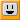這怎麼可能是真的？ 瀏覽1,184｜回應1｜推薦10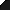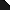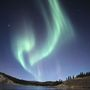渺忽隱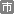等級：7 留言｜加入好友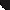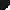文章推薦人 (10)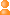Rosy雷尚淳yinghsuanJenny say阿彌陀佛!恰恰極墨歐陽玉敏煙‧新月‧就是瑜(小魚)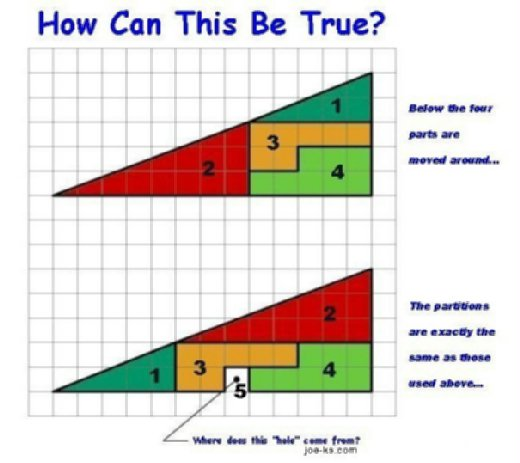引用網址：http://city.udn.com/forum/trackback.jsp?no=55869&aid=4254620回應文章 選擇排序方式 　最新發表 　最舊發表Answer is here! 推薦6渺忽隱等級：7 留言｜加入好友文章推薦人 (6)Rosy雷尚淳‧新月‧就是瑜(小魚)極墨煙

How Can This Be True - Answer

1. For both of the pictures to be exactly the same, they must both
have the same area AND each of the pictures must have same area as the
area of the "PERFECT" triangle (
Here are the calculated areas 4U:

Hint:
the area of a triangle = 1/2bh
where b is the base and h is the height

1A. Before looking at either picture, calculate what the area of this picture
would be if it was a PERFECT triangle (i.e. 13 X 5 squares)
Area = 1/2bh = 1/2(13)(5) = 65/2 = 32 1/2

1B. Area of the top picture
Area = 1 + 2 + 3 + 4

= 1/2(5)(2) + 1/2(8)(3) + 7 + 8
= 5 + 12 + 7 + 8 = 32

1C. Area of the lower picture
Area = 1 + 2 + 3 + 4 + 5

= 1/2(5)(2) + 1/2(8)(3) + 7 + 8 + 1
= 5 + 12 + 7 + 8 + 1 = 33

Neither of the two pictures form a perfect triangle,
but both of them look very close to the outline of a perfect triangle!

2. Compare the slopes of the triangles and you realize that
the hypotenuse of the overall triangle is not a straight line ...

Hint:
the slope of a line = rise / run
(the slope of a horizontal line is zero)

2A. Slope of the PERFECT triangle
Slope = rise / run = 5 / 13 = 0.384615

2B. Slope of Triangle 1 (in both pictures)
Slope = rise / run = 2 / 5 = 0.4

2C. Slope of Triangle 2 (in both pictures)
Slope = rise / run = 3 / 8 = 0.375

Prove it by blowing up the images and running a ruler along the
hypotenuse of both triangles - both are not a perfect line (but close!)

3. The bottom triangle has ~181.2 degrees when by definition a triangle must have only 180 degrees. I used the inverse tangent function and applied it to both angles that were not right angles. I calculated both what the angles should be based on the lengths of the whole triangle at the bottom and what they actually were based on the smaller triangles that formed the angles. The top right angle of the bottom triangle should be ~68.96 degrees based on tan^-1(13/5) but when you input the lengths of the smaller angle into this formula (tan^-1(8/3)) the angle comes out to be 69.44 degrees. Likewise with the other angle it should be ~21.04 degrees and actually is ~21.8. Larger angles mean more area into the shape.

Conclusion:  the 2 objects are NOT triangles
(even though they at first appear so!)
and they have different areas...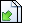引用網址：http://city.udn.com/forum/trackback.jsp?no=55869&aid=4256099